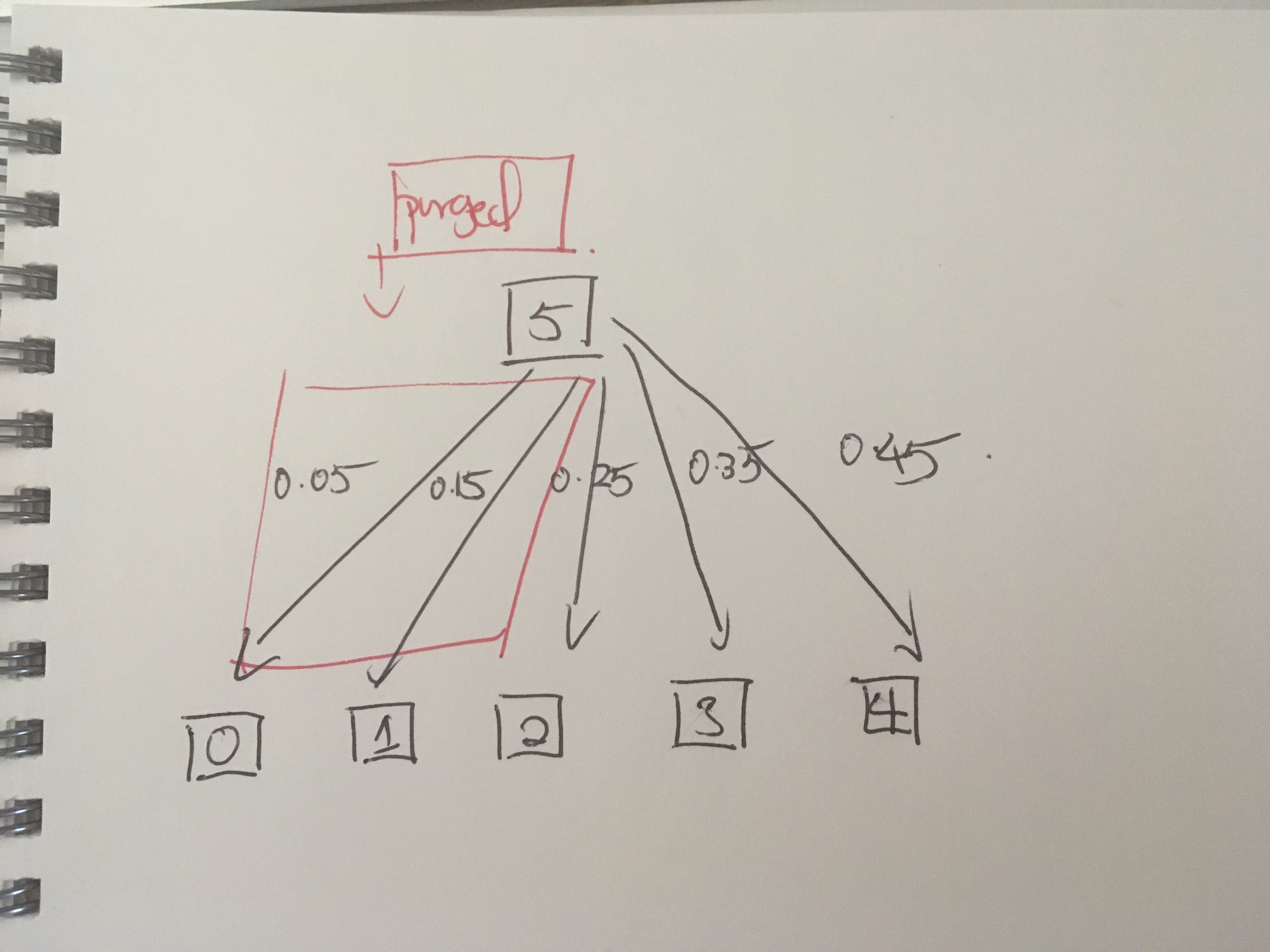# get_in_neighbours() and get_in_edges() output do not match

I have a directed graph:
<Graph object, directed, with 61560 vertices and 42858 edges, edges filtered
by (&lt;PropertyMap object with key type 'Edge' and value type 'bool', for
Graph 0x11a5e9190, at 0x11a5e96d0>, False), vertices filtered by
(<PropertyMap object with key type 'Vertex' and value type 'bool', for Graph
0x11a5e9190, at 0x11a5e9710>, False) at 0x11a5e9190>

I would like to get all in neighbors of a vertex, using the following codes:
for v in g.vertices():
print('')
print("vertex id:")
print(v)
print('all out neighbors')
print(g.get_out_neighbours(v))
print('all in neighbors')
print(g.get_in_neighbours(v))
print('all in edges')
print(g.get_in_edges(v))
raw_input()

Here's the console output:
vertex id:
0
all out neighbors

all in neighbors

all in edges
[[1 0 1]]

Output from get_in_edges() suggests that the in neighbor should be vertex 1;
but get_int_neighbours() suggests that the in neighbors is itself. I am not
sure what's going wrong. Any help would be appreciated. Thanks a lot!

Could you please provide a complete and minimal example that shows the
problem? (I.e. both the data *and* the code)

Best,
Tiago

Sure thing! This is the sample graph I've created, using the code at the
end of this message:

Here are a couple of observations:

1. get_in_neighbours() worked fine prior to purging the edges.
2. But once the edges are purged, the problem arises. Vertex 2, 3 and 4
should all have in neighbor 5, but g.get_in_neighbour(v) returns their own
vertex index.
3. get_in_edges() is working as intended.

Thanks a lot!

from graph_tool import *

# step 1: create a sample graph
g = Graph()

eprop_share = g.new_edge_property("double")
eprop_majorShare = g.new_edge_property("boolean")

i=0.05
for v in g.vertices():
eprop_share[e]=i
i=i+0..

# step 2: purge all edges with <0.2 share
for e in g.edges():
if eprop_share[e] >= 0.2:
eprop_majorShare[e] = True
else:
eprop_majorShare[e] = False

g.set_edge_filter(eprop_majorShare)
g.purge_edges()

# step 3: understand why get_in_neighbours and get_in_edges give
difference in neighbors
for v in g.vertices():
print('')
print("vertex id:")
print(v)
print('all out neighbors')
print(g.get_out_neighbours(v))
print('all in neighbors')
print(g.get_in_neighbours(v))
print('all in edges')
print(g.get_in_edges(v))
raw_input()

attachment.html (7.85 KB)Thanks for the example. As it turns out this is a bug that occurs only for
filtered graphs. I have now fixed it in git.

Best,
Tiago

You're welcome. Thanks for the update and this wonderful toolattachment.html (5.46 KB)